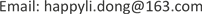1海军航空大学基础学院，山东 烟台

2中国人民解放军海军驻沈阳地区第一军事代表室，辽宁 沈阳

392728部队，上海1. 引言

2. 数值方法

{ ∂ ρ ∂ t + ∂ ∂ x i ( ρ u i ) = 0 ∂ ∂ t ( ρ u i ) + ∂ ∂ x j ( ρ u i u j ) + ∂ p ∂ x i = ∂ ∂ x j [ μ e f f ( ∂ u j ∂ x i + ∂ u i ∂ x j ) ] − 2 3 ∂ ∂ x j [ μ e f f ( ∂ u i ∂ x i ) ] ∂ ∂ t ( ρ E ) + ∂ ∂ x i ( ρ u i E ) + ∂ p u i ∂ x i = ∂ ∂ x j [ μ e f f u i ( ∂ u j ∂ x i + ∂ u i ∂ x j ) ] − 2 3 ∂ ∂ x j [ μ e f f u i ( ∂ u i ∂ x i ) ] + ∂ ∂ x j [ k e f f ( ∂ T ∂ x j ) ] p = ρ R T (1)

∂ ( l k ) ∂ t + ∂ ( l k u i ) ∂ x i = ∂ ∂ x j [ ( μ + μ t σ t ) ∂ k ∂ x j ] + G k + G b − l e − Y M + S k (2)

e方程是个由经验公式导出的方程：

∂ ( l e ) ∂ t + ∂ ( l e u i ) ∂ x i = ∂ ∂ x j [ ( μ + μ t σ e ) ∂ e ∂ x j ] + C 1 e e k ( G k + C 3 e G b ) − C 2 l e 2 k + S e (3)

3. 粗糙度的描述

1) 近于光滑壁面： 0 μm ≤ h s ≤ 5 μm ；

2) 过渡粗糙壁面： 5 μm ≤ h s ≤ 70 μm ；

3) 完全粗糙壁面： h s > 70 μm 。

u + = 1 × ln [ y + / ( 1 + 0.3 × k + ) ] / k + C (4)

k + = y R × ρ × u + / μ (5)

4. 模型的建立与求解4.1. 模型建立

4.2. 仿真计算

5. 仿真结果与分析

6. 结论

1) 叶片表面粗糙度的降低，这将导致压气机增压比和效率显著提高，扩大了压气机稳定工作范围，改善压气机总体工作特性，提高了压气机自身做功的能力，减少了流动损失。

2) 随着叶片表面粗糙度的降低，吸力面侧激波的位置向叶片出口方向逐渐移动，且强度减小明显，进一步使得叶片的尾迹区范围减小。

3) 随着叶片表面粗糙度的降低，叶片表面总温有不同程度的降低。当叶片粗糙度降低后，叶片表面总温的降低幅度从叶片底部到叶片顶部依次减小，随着转速降低依次减小。